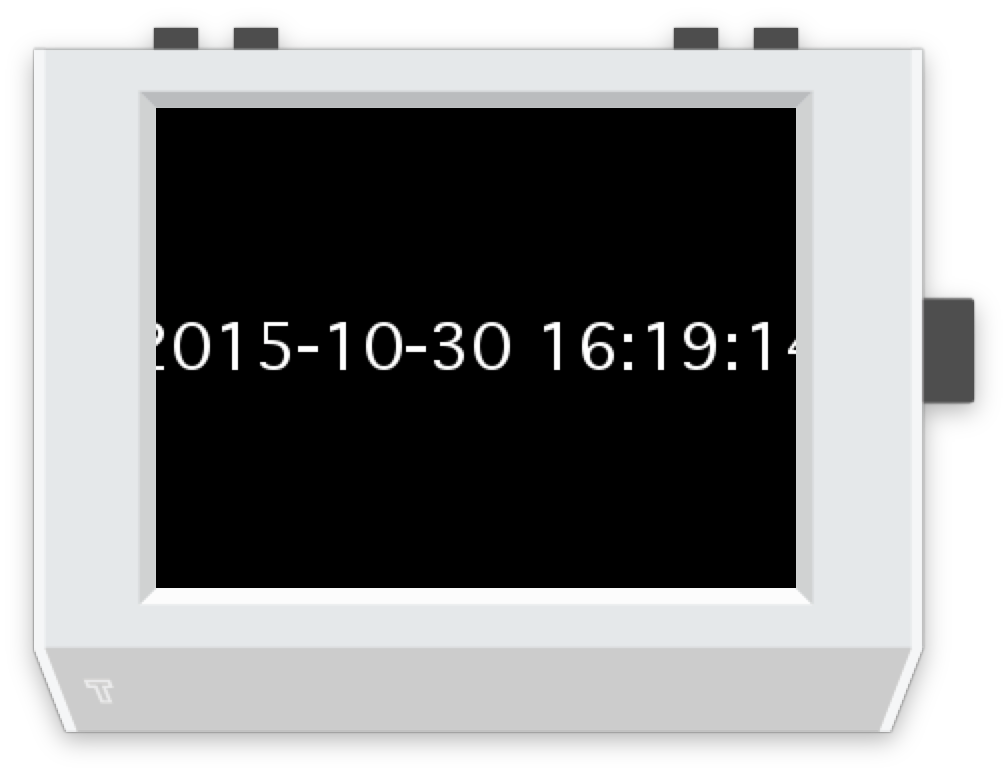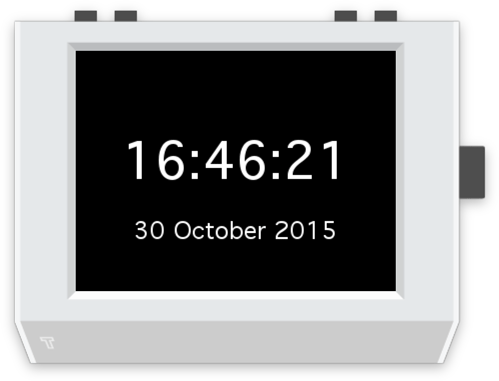# Getting started

## A clean slate

Open up Tide and choose File -> New.

You'll be presented with the app template, which looks like this:

``````import tingbot
from tingbot import *

# setup code here

@every(seconds=1.0/30)
def loop():
# drawing code here
screen.fill(color='black')
screen.text('Hello world!')

tingbot.run()
``````

This program just writes 'Hello world' to the screen. Try running it! Click the Run button in the top left of the Tide window to run this code in the simulator. It'll look like this:This program consists of a few parts-

### Import

``````import tingbot
from tingbot import *
``````

At the start, we import some functionality into the program. Here, we're importing everything from the tingbot libraries, so we can use `screen` to draw things to the screen. There are lots of things you can import, like `import requests` for getting data from the internet, or `import time` to access the system clock.

### Setup

``````# setup code here
``````

This part contains code that runs when your app starts. For this app, there's no setup required, so there's just a comment.

note

Comments begin with `#`, are used as notes-to-self, or notes to others reading this program, and have no effect on how the program runs.

### Loop

``````@every(seconds=1.0/30)
def loop():
# drawing code here
screen.fill(color='black')
screen.text('Hello world!')
``````

In this function, `loop()`, we draw to the screen. This function is run repeatedly while your program is running.

note

Functions are little bits of code that can be 'called' (meaning run) repeatedly from different parts of the program. This loop function is 'decorated' so it is called 30 times per second in the program.

### Run

``````tingbot.run()
``````

Finally we run the program. This line causes the `loop()` function to be called over and over, drawing to the screen.

## Make a change

Let's try making a change to our app. We can change the colors using the color parameter.

``````import tingbot
from tingbot import *

# setup code here

@every(seconds=1.0/30)
def loop():
# drawing code here
screen.fill(color='white')
screen.text('Hello world!', color='blue')

tingbot.run()
``````

## Your first app - a digital clock

For this app we're going to import some functionality from the Python time module.

``````import tingbot
from tingbot import *
import time

@every(seconds=1.0/30)
def loop():
current_time = time.strftime("%Y-%m-%d %H:%M:%S")

screen.fill(
color='black'
)
screen.text(
current_time,
color='white'
)

tingbot.run()
``````

A few things have changed in the above- we've removed the comments and split the `fill` and `text` calls to multiple lines. This is just to make it easier to read.

We've also added a call to `time.strftime`.

tip

Wondering where we got `time.strftime` from? The module `time` is part of Python, so it's always available, and you can learn about it from the Python docs.

We found it by Googling 'current time python' and checking the first search result!

So now instead of writing 'Hello world' to the screen, we're writing the current time. It looks like this:We're getting there!

note

Wondering what the `current_time` is? It's a variable. Variables are a way to carry data around in a program. So on the line `current_time = time.strftime("%Y-%m-%d %H:%M:%S")` we created and set the variable, and we used it in the `screen.text` call.

``````import tingbot
from tingbot import *
import time

@every(seconds=1.0/30)
def loop():
current_date = time.strftime("%d %B %Y")
current_time = time.strftime("%H:%M:%S")

screen.fill(
color='black'
)
screen.text(
current_time,
xy=(160, 110),
color='white',
font_size=50,
)
screen.text(
current_date,
xy=(160, 180),
color='white',
font_size=24,
)

tingbot.run()
``````